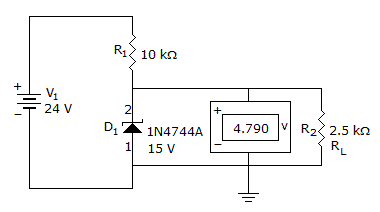# Electronics - Diodes and Applications

### Exercise :: Diodes and Applications - General Questions

36.

What is the percent of regulation if Vnl = 20 V and Vfl = 19.8 V?

 A. 0% B. 1% C. .1% D. 5%

Answer: Option B

Explanation:

No answer description available for this question. Let us discuss.

37.

With a half-wave rectified voltage across the load resistor, load current flows for what part of a cycle?

 A. 0 degrees B. 90 degrees C. 180 degrees D. 360 degrees

Answer: Option C

Explanation:

No answer description available for this question. Let us discuss.

38.

Which of the following circuits would require the least amount of filtering?

 A. A half-wave rectifier B. A full-wave rectifier C. A bridge rectifier D. A full-wave rectifier and a bridge rectifier

Answer: Option D

Explanation:

No answer description available for this question. Let us discuss.

39.

What is wrong with this circuit?A. The zener is open. B. The zener is shorted. C. nothing D. not enough data

Answer: Option A

Explanation:

No answer description available for this question. Let us discuss.

40.

The voltage where current may start to flow in a reverse-biased pn junction is called the

 A. breakdown voltage B. barrier potential C. forward voltage D. biasing voltage

Answer: Option A

Explanation:

No answer description available for this question. Let us discuss.

#### Current Affairs 2021

Interview Questions and Answers